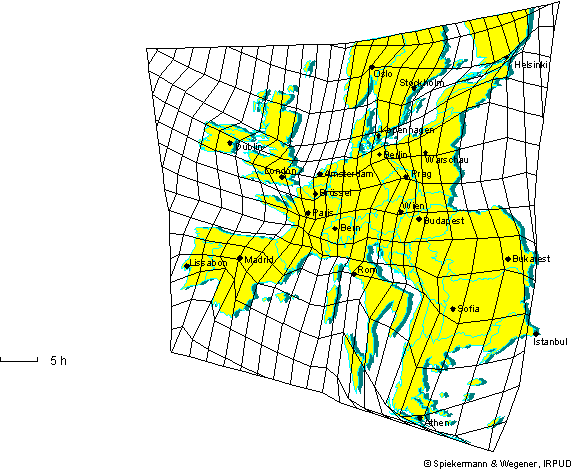iSoul In the beginning is reality.

# Introduction to duration space

Since Newton, time has been the usual and ultimate independent variable for physics. This contrasts with problems in transportation, where time is often optimized. Whether transporting goods across the world, commuters across town, or athletes to the finish line, length is the independent variable against which time is measured and optimized. If length is taken as the independent variable for physics, a mechanics results that is different from Newton’s but equivalent to it. In what follows we explore the basic kinematics and dynamics with length as the independent variable, first in classical, then in relativistic mechanics.

To represent time on a map, one may use isochrones (time contours), as in this map of tsunami travel time in hours:Or time may be used instead of length, as in this map of European rail travel times in hours:Note that in this map it is time (duration) that is two dimensional, not length space. It looks like a distortion of the length space representation, but it is not a distortion. It is a time map with units of duration rather than units of length. The multiple dimensions of duration will be a main feature of taking distance as the independent variable.

Length could be included as a distance from a particular place, represented by an iso-distance contour. That is, length is reduced to one dimension if time is the dependent variable. 1D length space is a baseline.

Units such as natural units may be adopted to equalize the units for length and time, i.e., by adopting a constant modal speed dependent on the mode of travel. However, they are still different measurements. Such units allow constant speeds to be represented the same, whether in base or time, which minimizes the seeming distortion of a time map.

As I pointed out here, an independent variable is given to us and so not in our control, and so may seem to flow on independently. A clock is like that, and it gives us a sense that time flows. But a stopwatch starts and stops at our command. Time no longer flows.

Distance can seem to flow if we allow it to. Consider a hop-on, hop-off transit system with a fixed route. It cycles through its various destinations and then repeats the cycle. The distance between two stops may be read from an odometer by subtracting the earlier from the later distance. This is just like finding the time between two points with a clock. Only here it’s the transit system that flows on, accumulating distance indefinitely. Distance flows instead of time.